# 空化水动力学非定常特性研究进展及展望

1 武汉大学水资源与水电工程科学国家重点实验室, 武汉 430072
2 北京理工大学机械与车辆学院, 北京 100081
3 清华大学水沙科学与水利水电工程国家重点实验室, 北京 100084
4 中国船舶科学研究中心船舶振动噪声重点实验室, 江苏无锡 214082;

# Research progresses and prospects of unsteady hydrodynamics characteristics for cavitation

JI Bin1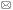, CHENG Huaiyu1, HUANG Biao2, LUO Xianwu3, PENG Xiaoxing4, LONG Xinping1

1 State Key Lab of Water Resources and Hydropower Engineering Science,Wuhan University, Wuhan 430072,China
2 School of Mechanical Engineering, Beijing Institute of Technology, Beijing 100081, China
3 State Key Lab of Hydroscience & Engineering, Tsinghua University,Beijing 100084, China;
4 National Key Lab on Ship Vibration and Noise, China Ship Scientific Research Center, Wuxi 214082, Jiangsu, China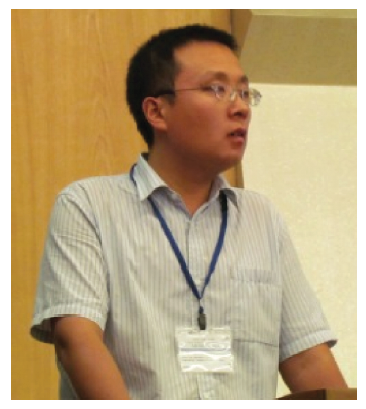Abstract

Cavitation is an important kind of complex multiphase flow with strong three-dimensional characteristic and high unsteadiness, which frequently occurred in a wide range of fluid machinery, marine propulsor, and hydraulic engineering and can generate the destructive behavior. Cavitation has been one of the most difficult and key problems in the area of hydrodynamics for quite a long time. In this paper, the research progress of unsteady hydrodynamics characteristics for cavitation is reviewed from the viewpoints of experimental and numerical investigations, respectively. And the existing problems in the cavitation research are also discussed. For the experimental study, the progress of the cavitation mechanism tunnel, measurement technology for cavitating flow and simultaneous sampling technique are introduced. For the numerical investigations, some of the most popular cavitation models and turbulence models are introduced by categorization, and the applications of large eddy simulation (LES) approach and validation & verification in cavitation simulations are discussed in detail. Then, mainly based on attached cavity but also other kinds of cavitation, such as cavitation cloud, cavitation erosion, and vortex cavitation, several basic but important problems are discussed. Problems discussed herein includes the evolution of attached cavity, the three dimensional structures of cavitation, the shedding mechanism of attached cavity, the unsteadiness mechanism of cavitation and its connection with the pressure fluctuations, the interaction between cavitation and vortex, the fluid-structure interaction in the cavitating flow around a flexible hydrofoil, influence of cavitation on the wake flow, and so on. Finally, prospects of the direction and trends of cavitation hydrodynamics research are discussed.

Keywords： cavitation ; hydrodynamics ; cavitating flow ; attached cavitation ; cavitation model

0

JI Bin, CHENG Huaiyu, HUANG Biao, LUO Xianwu, PENG Xiaoxing, LONG Xinping. Research progresses and prospects of unsteady hydrodynamics characteristics for cavitation[J]. Advances in Mechanics, 2019, 49(1): 201906-201906 https://doi.org/10.6052/1000-0992-17-012

## 1 引 言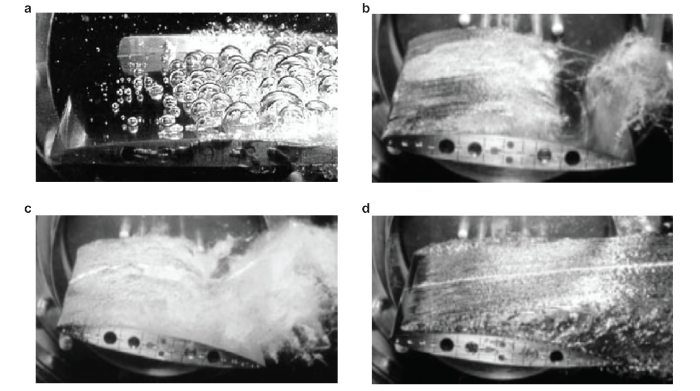## 2 空化实验平台与实验技术进展

### 2.1 空化实验平台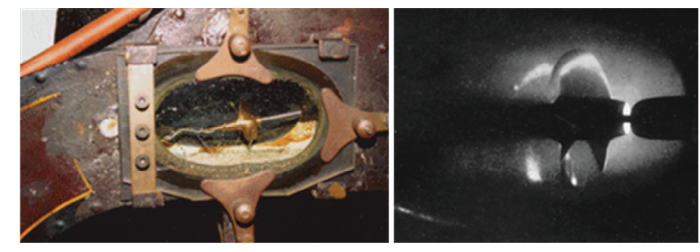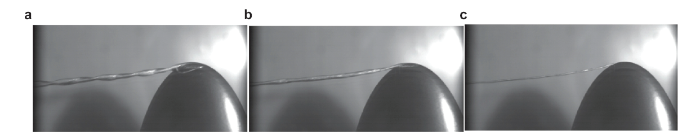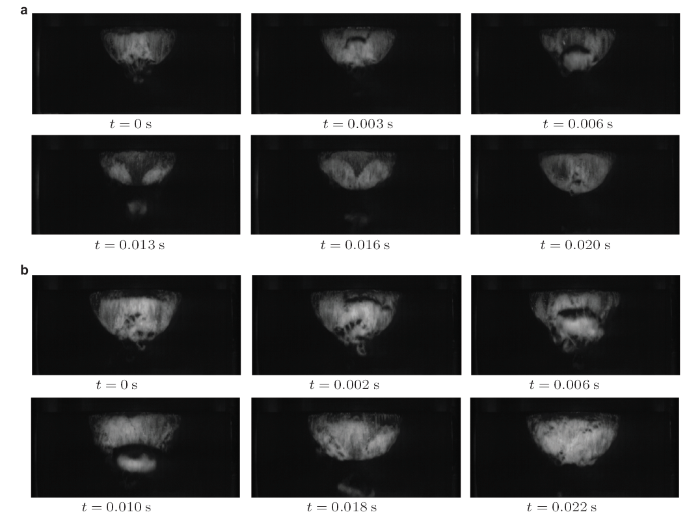### 2.3 空化流动速度场测量技术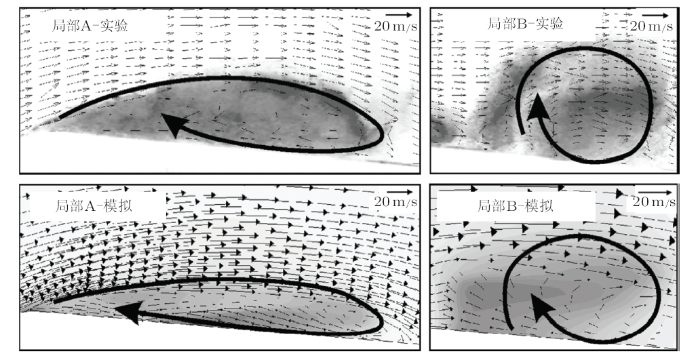### 2.4 空化流动蒸汽含量测量技术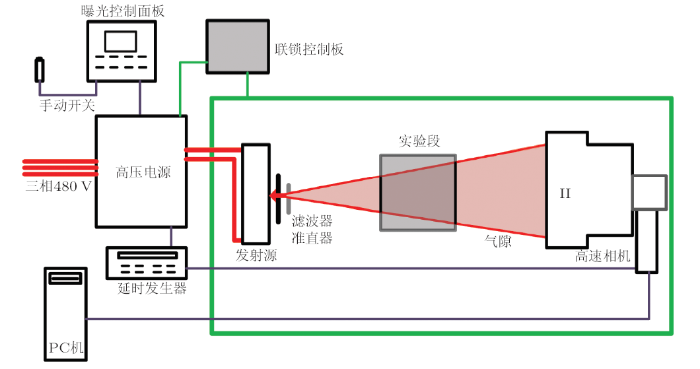### 2.5 多物理场同步测量技术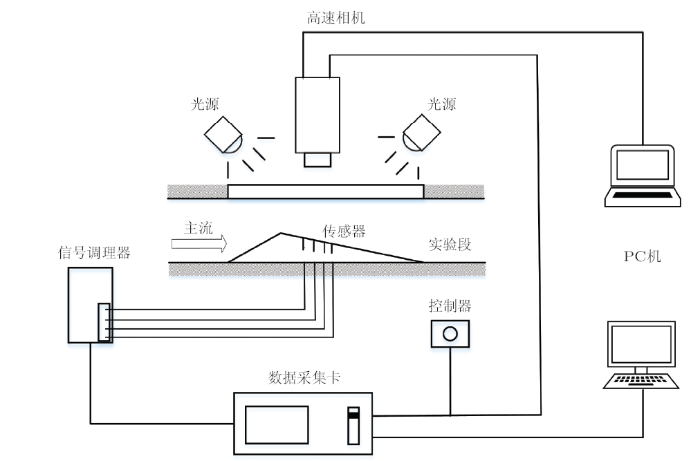## 3 空化数值模拟方法的进展

### 3.1 常用的几种空化模型

3.1.1 基于正压流体状态方程的空化模型

$$\rho _{\rm m} =f(p)(1)$$

$$\Delta p_{\rm v} = \pi c_{\min }^2 \dfrac{\rho _{\rm l} - \rho _{\rm v} }{2}(2)$$

$$\rho _{\rm m} = f(p) = \left\{ \begin{array}{ll} \rho _{\rm ref} \left( {\dfrac{p + p_0 }{p_{\rm ref}^{\rm T} + p_0 }} \right)^{1 / n}, &\ p > p_{\rm v} + 0.5\Delta p_{\rm v} \\ \dfrac{\rho _{\rm l} + \rho _{\rm v} }{2} + \dfrac{\rho _{\rm l} - \rho _{\rm v} }{2}\sin \left( {\pi \cdot \dfrac{p - p_{\rm v} }{\Delta p_{\rm v} }} \right), &\ p_{\rm v} - 0.5\Delta p_{\rm v} < p < p_{\rm v} + 0.5\Delta p_{\rm v} \\ \dfrac{p}{RT} , &\ p < p_{\rm v} - 0.5\Delta p_{\rm v} \\ \end{array} \right.(3)$$

3.1.2 基于质量输运方程的空化模型

$$\dfrac{\partial (\rho _{\rm v} \alpha _{\rm v} )}{\partial t} + \dfrac{\partial (\rho _{\rm v} \alpha _{\rm v} u_i )}{\partial x_{\rm i} } = \dot {m}^ + - \dot {m}^ -(4)$$